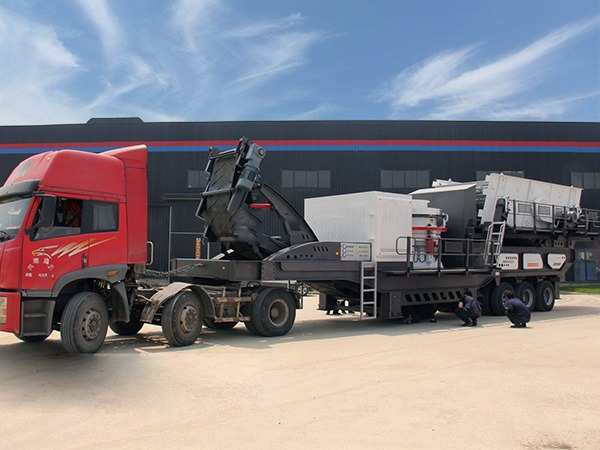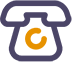# 移动破碎站

【应用范围】：矿山、煤矿、垃圾及建筑垃圾循环再利用、土石方工程、城市基础设施、道路或建筑工地等场地作业。

【适用物料】：河卵石、山石（石灰石、花岗岩、玄武岩、辉绿岩、安山岩等）、矿石尾矿等。24小时全国咨询热线

• 主机采用新型建筑垃圾专用破碎机，可以破碎可循环回收利用的物料，以及软物料、中等硬度物料；
• 破碎比大，进料粒度大；

产量高，产品粒型优异，磨损成本低；
• 独特的破碎腔型设计，能够对建筑垃圾提供有效的破碎；
• 具有环保性，包括基本的降尘和降噪配置。

### 技术参数

 型号 给料机型号 破碎机型号 主皮带机型号 侧出皮带机型号 最大进料 生产能力(t/h) 电机功率(kw) 除铁器(选配) 轮胎配置 YPS150 GZT-0724 PJ1108 B500=7m B650=8m 600 40-100 125.5 RCYD 双轴 YPS200 GZT-0932 PJ1110 B500=3m B650=8m 700 60-150 172.5 RCYD 双轴 YPS260 GZT-380x96 PJ1113 B800=8m B650=8m 800 100-200 196 RCYD 双轴

 型号 振动筛型号 给料皮带机型号 返料皮带机型号1 出料皮带机型号 出料皮带机型号 出料皮带机型号 功率 轮胎配置 YPS150 YA1545 B650=11.8mm B650=6.5m B650=8m B500=3m B500=3m 37.5KW 双轴 YPS200 3YA1860 B650=11.8mm B650=6.5m B650=5.5m B500=2m B500=3m 39.5KW 双轴 YPS260 3YA1860 B650=11.8mm B650=7.5m B650=5.5m B500=2m B500=5m 67KW 双轴